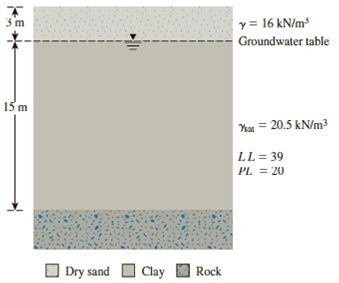Chapter 12, Problem 12.24PPrinciples of Geotechnical Enginee...

9th Edition
Braja M. Das + 1 other
ISBN: 9781305970939

Solutions

Chapter
SectionPrinciples of Geotechnical Enginee...

9th Edition
Braja M. Das + 1 other
ISBN: 9781305970939
Textbook Problem

Refer to the clay soil in Figure 12.55. If the natural moisture content is 28%, estimate the undrained shear strength of remolded clay using the relationships given in Table 12.5 bya. Leroueil et al. (1983)b. Terzaghi et al. (1996)Figure 12.55

(a)

To determine

Find the undrained shear strength of remolded clay using the relationships Leroueil et al.

Explanation

Given information:

The natural moisture content (w) is 28%.

The liquid limit (LL) of the clay is 39.

The plastic limit (PL) of the clay is 20.

Calculation:

Find the Liquidity index (LI) using the relation.

LI=wPLLLPL (1)

Substitute 39 for LL, 28% for w, and 20 for PL in Equation (1).

LI=28203920=819=0.421

Find the undrained shear strength (cur) of remolded clay using equation Leroueil et al (1983).

(cur)=1[(LI)0

(b)

To determine

Find the undrained shear strength of remolded clay using the relationships Terzaghi et al.(1996).

Still sussing out bartleby?

Check out a sample textbook solution.

See a sample solution

The Solution to Your Study Problems

Bartleby provides explanations to thousands of textbook problems written by our experts, many with advanced degrees!

Get Started

Find more solutions based on key concepts